## Zero

Sunday 29 February 2004 at 12:48 pm | In Articles | Post Comment
1. Why is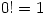?
2. By looking atand atwhat can you say about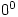?
3. Can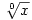(the zeroth root of x) be defined? If so, how; if not, why?
4. Criticise the following ‘proof’: If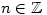then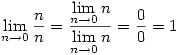## Powers and Logs

Monday 23 February 2004 at 8:10 pm | In Articles | Post Comment

Prove that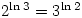The proof should show you how this generalises. If you have studied group theory you can extend this even further by showing that, where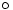is defined by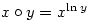, is an abelian group.
Why can’t 1 be an element of this group?

## Factorials

Saturday 21 February 2004 at 11:06 pm | In Articles | Post Comment

Inspired by a test posting on S.O.S. Mathematics CyberBoard

Factorials are fascinating. They are obtained by multiplying the numbers 1, 2, 3, 4, 5 … together and are written using the ! sign. ThusFactorials get large very quickly so 25! = 15 511 210 043 330 985 984 000 000 and as it gets larger there are more and more zeros.

Question: How can you find the number of zeros in 102! without calculating it?
Answer: All you do is count the number of times powers of 5 divide into 102 and add them up. So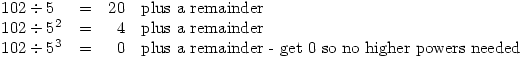Throw away the remainders and we get that there are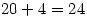zeros in 102!
Now find how many zeros there are in 2004!

Can you show why this method works? Can you generalise it? Have a go, then look for answers on the internet.

Mathematicians will recognise this as a special case of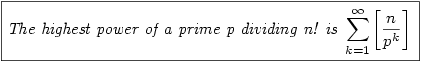which is not difficult to prove by induction.

More on factorials at mathworld

## Two Problems

Sunday 15 February 2004 at 3:34 pm | In Articles | Post Comment

2. If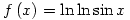find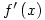[Hint: think carefully about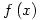before you differentiate]